Home > Products > Employee Survey > Articles > T-Scores Explained

# Employee Survey Benchmarks: Understanding T-Scores

 Tweet

Employee satisfaction or engagement survey results are typically presented in two different ways - as raw scores and as benchmark scores.

Raw Scores are simply the average of all responses. Survey questions that measure employee satisfaction or engagement usually use a 5-point rating scale, where the lowest possible raw score is a 1, and the highest possible raw score is a 5.

By themselves, raw scores don't tell you whether a score is high or low. How people respond to a survey question depends on how the question is worded, as explained here. For example, on one survey question, a raw score of 3.6 might be considered a high score, but that same score on another survey question might be quite low.

When looking at employee satisfaction or engagement survey results, you can't draw conclusions about levels of satisfaction or engagement by just looking at raw scores. If you want to know whether a score is good or bad, you need to understand how your results compare to the results received by other organizations. In other words, you need some way to benchmark your survey results.

T-Scores are one way of representing benchmark scores. Like all benchmarking methods, T-Scores eliminate the natural variations between survey questions and provide a way to determine whether scores are high or low.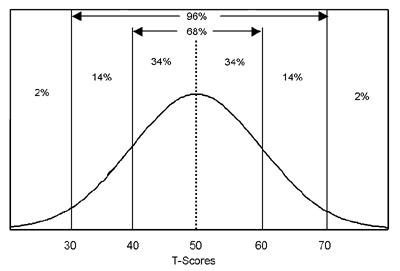T-Scores tell you how your scores compare to the scores received by other organizations.

When using T-Scores, the mean (average) is always 50, and the standard deviation is 10. If your T-Score is above 50, then it is above average. If your score is less than 50, it is below average. For example, if you have a T-Score of 60, you scored higher than 84% of all organizations.

T-Scores are distributed in a bell-shaped curve, as illustrated in the diagram to the right. 68% of T-Scores fall between 40 and 60. 96% of scores fall between 30 and 70. Only 4% of scores fall below 30 or above 70.
In order to calculate T-Scores, we need to start with good normative (benchmark) data. This is obtained by collecting survey responses from employees at hundreds of different organizations. We then calculate the average (mean) and the standard deviation of the scores for each survey question across all those organizations. (Standard deviation is a measure of variation. It indicates how much scores vary across different organizations.)

To calculate a T-Score, we take your organization's raw score minus the normative mean (average across all organizations) and divide by the standard deviation. Lastly, we multiply by 10 and add 50. (These last steps simply transform the score into a range that is more intuitive. If we omit these last steps, the score is referred to as a z-score, with an average of zero and a standard deviation of 1.)

In other words:
T-Score = (Your Organization's Raw Score - Average Score) / Standard Deviation * 10 + 50

Examples of T-Score Calculations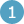Sample survey question: "Employee satisfaction is a priority in this company." Your organization's raw score: 4.2 (for example) Average score across all organizations: 3.7 Standard deviation across all organizations: 0.5 T-Score = (4.2 - 3.7) / 0.5 * 10 + 50 = 60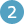Sample survey question: "Employee satisfaction is the top priority in this company." Your organization's raw score: 3.6 (for example) Average score across all organizations: 3.1 Standard deviation across all organizations: 0.5 T-Score = (3.6 - 3.1) / 0.5 * 10 + 50 = 60

These are just examples, but they also illustrate how benchmark scores provide essential context to raw scores. The two sample survey questions are worded differently, which influences how people respond to them. Notice how the raw scores are very different but lead to identical T-Scores.

Why and how does benchmarking work?
When we look at engagement or satisfaction survey results across a large number of different organizations, we find that scores on survey questions tend to follow a normal distribution. This means that scores follow a fairly predictable bell-curve pattern.

The chart below is an example of how different companies scored on a survey question that measures employee satisfaction.

The vertical axis indicates the number of companies, and the horizontal axis indicates the score. For example, 20 companies received a score of 3.1.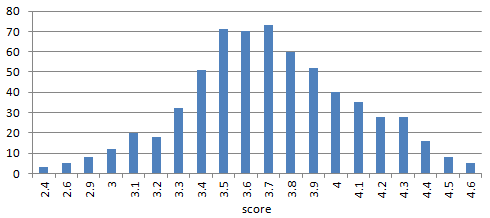Notice how the response distribution approximates a bell-curve shape. It is not a perfect fit, but it is close.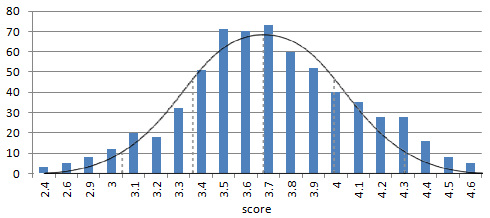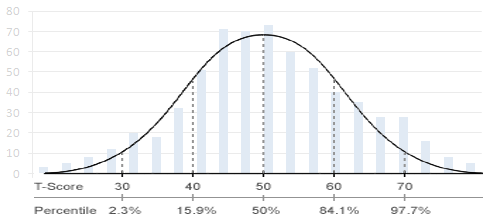This tells us that the responses to this survey question are "normally distributed". Since the responses are normally distributed, we can use benchmark scores to describe how high or low any particular company's score is compared to other companies.

As noted above, we use T-Scores to describe how high or low a score is. Another way to represent benchmark scores is with percentiles.

To visually understand how a benchmark score works, pick a score from one chart and see what the equivalent T-Score or percentile score is.

For example, on this chart, a score of 3.4 equates to a T-Score of approximately 41 or the 18th percentile.

Popular Employee Engagement Article Topics and Resources:

 Tweet